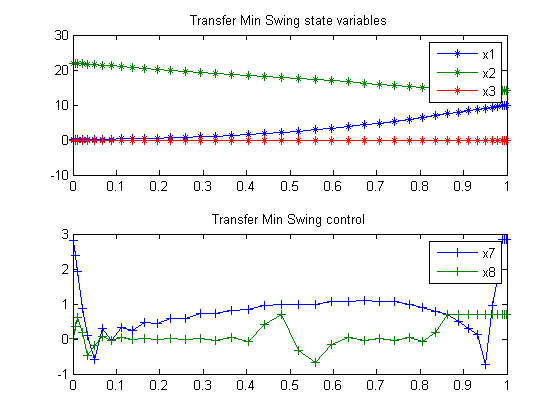117  Transfer Min Swing

Example 7.1: K.L. Teo, K. K. Leong, G.J. Goh

117.1  Problem Formulation

Find u over t in [0; 1 ] to minimize

J =
 1 0
4.5 (x32 + x62dt

subject to:

 dx1 dt
= 9*x4
 dx2 dt
= 9*x5
 dx3 dt
= 9*x6
 dx4 dt
= 9*(x7+17.2656*x3
 dx5 dt
= 9*x8
 dx6 dt
= −9*
 x7+27.0756*x3+2*x5*x6 x2

 dx7 dt
= 9*u1
 dx8 dt
= 9*u2

 x(0) = [0  22  0  0  −1  0  NaN  NaN]
 x(1) = [10  14  0  2.5  0  0  NaN  NaN]
 |x4| <= 2.5
 |x5| <= 1.0
 |x7| <= 2.83374
 −0.80865 <= x8 <= 0.71265
 |u| <= 10

Reference: 

toms t phi1 phi2

% Starting guess
speed = 5;
xopt = 1.2*t;
yopt = 1.6*t;
thetaopt = pi/4;

phi1opt = 1;
phi2opt = 1;
x1opt = 10*t;
x2opt = 22-8*t;
x3opt = 0;
x4opt = 2.5*t;
x5opt = -1+t;
x6opt = 0;
x7opt = 0;
x8opt = 0;
u1opt = 0;
u2opt = 0;

117.3  Solve the problem, using a successively larger number collocation points

for n=[20 40]
% Create a new phase and states, using n collocation points
p = tomPhase('p', t, 0, 1, n);
setPhase(p);
tomStates x1 x2 x3 x4 x5 x6 x7 x8
tomControls u1 u2

% Initial guess
x0 = {phi1 == phi1opt; phi2 == phi2opt
icollocate({
x1 == x1opt; x2 == x2opt
x3 == x3opt; x4 == x4opt
x5 == x5opt; x6 == x6opt
x7 == x7opt; x8 == x8opt})
collocate({
u1 == u1opt; u2 == u2opt})};

% Box constraints
cbox = {-10  <= phi1 <= 10
-10      <= phi2 <= 10
-2.5     <= icollocate(x4) <= 2.5
-1       <= icollocate(x5) <= 1
-2.83374 <= icollocate(x7) <= 2.83374
-0.80865 <= icollocate(x8) <= 0.71265
-10      <= collocate(u1) <= 10
-10      <= collocate(u2) <= 10};

% Boundary constraints
cbnd = {initial({x1 == 0
x2 == 22; x3 == 0
x4 == 0;  x5 == -1
x6 == 0;  x7 == phi1
x8 == phi2
})
final({x1 == 10
x2 == 14;  x3 == 0
x4 == 2.5; x5 == 0
x6 == 0})};

% ODEs and path constraints
ceq = collocate({dot(x1) == 9*x4
dot(x2) == 9*x5; dot(x3) == 9*x6
dot(x4) == 9*(x7+17.2656*x3)
dot(x5) == 9*x8
dot(x6) == -9*(x7+27.0756*x3+2*x5.*x6)./x2
dot(x7) == 9*u1; dot(x8) == 9*u2});

% Objective
objective = integrate(4.5*(x3.^2 + x6.^2));

117.4  Solve the problem

options = struct;
options.name = 'Transfer Min Swing';
solution = ezsolve(objective, {cbox, cbnd, ceq}, x0, options);

% Optimal x and u to use as starting guess in the next iteration
x1opt = subs(x1, solution);
x2opt = subs(x2, solution);
x3opt = subs(x3, solution);
x4opt = subs(x4, solution);
x5opt = subs(x5, solution);
x6opt = subs(x6, solution);
x7opt = subs(x7, solution);
x8opt = subs(x8, solution);
u1opt = subs(u1, solution);
u2opt = subs(u2, solution);
phi1opt = subs(phi1, solution);
phi2opt = subs(phi2, solution);
Problem type appears to be: qpcon
Starting numeric solver
===== * * * =================================================================== * * *
TOMLAB - Tomlab Optimization Inc. Development license  999001. Valid to 2011-02-05
=====================================================================================
Problem: ---  1: Transfer Min Swing             f_k       0.005155677076381509
sum(|constr|)      0.000000000219058091
f(x_k) + sum(|constr|)      0.005155677295439600
f(x_0)      0.000000000000000000

Solver: snopt.  EXIT=0.  INFORM=1.
SNOPT 7.2-5 NLP code
Optimality conditions satisfied

FuncEv    1 ConstrEv  107 ConJacEv  107 Iter  100 MinorIter  375
CPU time: 0.515625 sec. Elapsed time: 0.547000 sec.
Problem type appears to be: qpcon
Starting numeric solver
===== * * * =================================================================== * * *
TOMLAB - Tomlab Optimization Inc. Development license  999001. Valid to 2011-02-05
=====================================================================================
Problem: ---  1: Transfer Min Swing             f_k       0.005157874717791312
sum(|constr|)      0.000000001283862156
f(x_k) + sum(|constr|)      0.005157876001653469
f(x_0)      0.005155729096774477

Solver: snopt.  EXIT=0.  INFORM=1.
SNOPT 7.2-5 NLP code
Optimality conditions satisfied

FuncEv    1 ConstrEv  126 ConJacEv  126 Iter  123 MinorIter  594
CPU time: 2.453125 sec. Elapsed time: 2.578000 sec.
end

t  = subs(collocate(t),solution);
x1 = collocate(x1opt);
x2 = collocate(x2opt);
x3 = collocate(x3opt);
x7 = collocate(x7opt);
x8 = collocate(x8opt);

117.5  Plot result

subplot(2,1,1)
plot(t,x1,'*-',t,x2,'*-',t,x3,'*-');
legend('x1','x2','x3');
title('Transfer Min Swing state variables');

subplot(2,1,2)
plot(t,x7,'+-',t,x8,'+-');
legend('x7','x8');
title('Transfer Min Swing control');